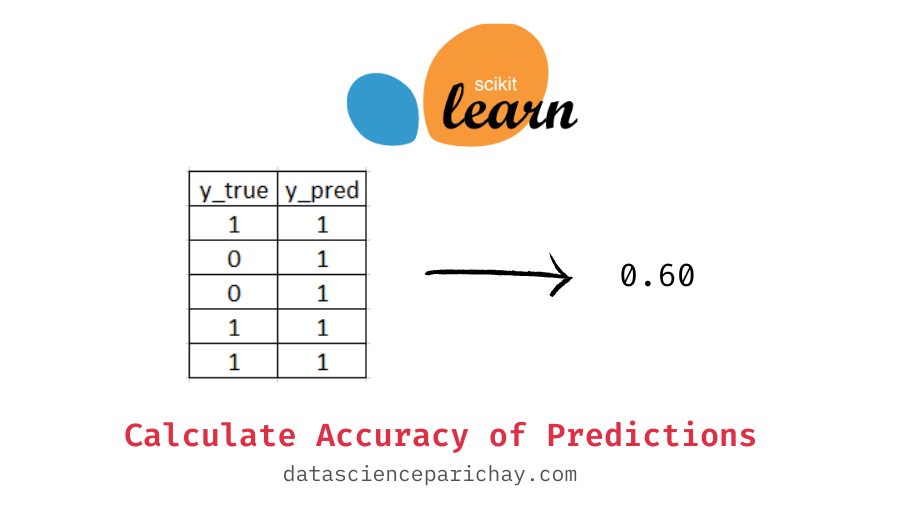# Get Accuracy of Predictions in Python with Sklearn

Measuring the performance of your model using the correct metric is a very important step in the data science process. In this tutorial, we’ll look at how to compute the accuracy of your predictions from scratch and with sklearn in Python.

Accuracy is one of the most common metrics used to judge the performance of classification models. Accuracy tells us the fraction of labels correctly classified by our model. For example, if out of 100 labels our model correctly classified 70, we say that the model has an accuracy of 0.70

Let’s write a function in python to compute the accuracy of results given that we have the true labels and the predicted labels from scratch.

```def compute_accuracy(y_true, y_pred):
correct_predictions = 0
# iterate over each label and check
for true, predicted in zip(y_true, y_pred):
if true == predicted:
correct_predictions += 1
# compute the accuracy
accuracy = correct_predictions/len(y_true)
return accuracy```

The above function takes in values for the true labels and the predicted labels as arguments and returns the accuracy score. Here, we count the total number of correct predictions by iterating over each true and predicted label combination in parallel and compute the accuracy by dividing the number of correct predictions by the total labels.

Let’s try the above function on an example.

```# sample labels
y_true = [1, 0, 0, 1, 1]
y_pred = [1, 1, 1, 1, 1]
# get the accuracy
compute_accuracy(y_true, y_pred)```

Output:

`0.6`

We get 0.6 as the accuracy because three out of five predictions are correct.

Note that, the above function can be optimized by vectorizing the equality computation using numpy arrays.

📚 Data Science Programs By Skill Level

Introductory

Intermediate ⭐⭐⭐

🔎 Find Data Science Programs 👨‍💻 111,889 already enrolled

Disclaimer: Data Science Parichay is reader supported. When you purchase a course through a link on this site, we may earn a small commission at no additional cost to you. Earned commissions help support this website and its team of writers.

You can also get the accuracy score in python using sklearn.metrics’ accuracy_score() function which takes in the true labels and the predicted labels as arguments and returns the accuracy as a float value. `sklearn.metrics` comes with a number of useful functions to compute common evaluation metrics. For example, let’s compute the accuracy score on the same set of values as above but this time with sklearn’s accuracy_score() function.

```from sklearn.metrics import accuracy_score
accuracy_score(y_true, y_pred)```

Output:

`0.6`

You can see that we get an accuracy of 0.6, the same as what we got above using the scratch function. It is recommended that you use the sklearn’s function as it not only is optimized for performance but also comes with additional parameters that might be helpful.

For more on the sklearn’s accuracy_score() function, refer to its documentation.

With this, we come to the end of this tutorial. The code examples and results presented in this tutorial have been implemented in a Jupyter Notebook with a python (version 3.8.3) kernel having numpy version 0.23.1

•